### File information

This PDF 1.5 document has been generated by / Skia/PDF m55, and has been sent on pdf-archive.com on 06/10/2016 at 18:29, from IP address 209.221.x.x. The current document download page has been viewed 427 times.
File size: 552.79 KB (8 pages).
Privacy: public file

### Document preview

RHHS Compsci 2016

Week 2 - October 6th

REVIEW
Do you still remember stuff from last week?

USING IDLE
CONSOLE
When you first launch IDLE, you will be in interactive mode.
This above image is what you will see, where you can enter a
single line of a command, and it will run immediately, without
any need to save or compile.
In interactive mode, you can only run single lines of code.

EDITOR
You have to be editing a file to be in scripting mode. This mode
lets you enter multiple lines of code, and you will have to save
it as a .py before you can run it. When you run the program, it
will open a new window. You can find the run command
under the “Run” menu, followed by “Run module,” or simply

VARIABLES
Variables are essentially locations in memory that ​store your
data​. They can be accessed and modified as many times as
you want. To declare a variable, simply state the name you want to use for your variable and
assign it data using the ​= sign. For example, a​ ge = 16​. Make sure the ​variable name is on the
left and the value on the right​. To change or access it later on, just declare the variable again
with whatever you want to change the value to. (ex. ​age = age + 1​)
It is important to write descriptive variable names so that in larger programs the function of a
variable is very clear to the programmer when looking back. (​ex. noOfTurkeys as opposed to
turks​)

Camel Case
This example has two variables declared. (These
will be explained soon.) Note how the difference in
capitalization in the variable names produces two
different variables. Also, note how the capitalization
is done. The first is in something called “​camelCase​.”
In camel case, we don’t use spaces (variable names
can’t have spaces in them), so we separate multiple
words in the name by capitalizing each first letter

RHHS Compsci 2016

except in the first word. (​ex. thisIsTheNameOfMyVariable​)
It is against convention to capitalize the first word in a variable name. Underscores can also
be used in order to ​SEPARATE_CAPITALS_IN_VARIABLE_NAMES​.
There are also some other restrictions on variable names, such as ​reserved words​. Reserved
words are words such as “​break​”, “​continue​”, “​range​”, and so on. These are special words that
Python interprets differently and can make the program behave in a special way.

Arithmetic
Arithmetic is pretty similar to regular math, but you
just have to remember to assign the answer from
the operation to a variable. Yes, you can make your
Python program do the math for you, like a
calculator. There are also many operations you can
do in Python that your calculator can’t do. Note that
valueOne, valueTwo, etc. can be any numbers or
number variables. Where noted, remember to write
import math and/or ​import random ​at the
&gt;​Subtract:​ valueOne - valueTwo
&gt;​Multiply:​ valueOne * valueTwo
&gt;​Divide:​ valueOne / valueTwo
Note: Division will always result in a float, even
when the quotient is a whole number.
&gt;​Integer Divide: valueOne // valueTwo ​(Divides,
then only gives you back the digits before the
decimal point. ​3 // 2 = 1)
&gt;​Modulus: valueOne % valueTwo ​(Finds the
remainder of the division)
&gt;​Power:​ baseNumber ** exponent
ex. 2**3 is 23​
&gt;​Absolute value: abs(valueOne) ​(Makes the number positive. ​abs(-2) = 2
and ​abs(2) = 2)
&gt;​Min/max: max(valueOne, valueTwo, valueThree, etc.) and
min(valueOne, valueTwo, etc.) ​(Find the highest or lowest value in a set of
numbers, respectively.)
&gt;​Round: round(valueOne, chosenDecimal) ​(Rounds to the nearest chosen
decimal, 0 being whole number)
&gt;​Random: random.randint(min,max) ​(Chooses a random integer from min to max) *
Remember to ​import random
&gt;​There are plenty more math functions you can find online

RHHS Compsci 2016

Basic output
The print command is the most basic form of outputting information to the screen. We will
move onto graphical output later on. Here are some examples of how to use it:
&gt;print(“text”)
This command simply displays the given text within
the parenthesis. Remember to put quotation marks
around raw text (ex. ​print(“Hello World”)​) as opposed
to print(Hello World), because it will otherwise look
for variables named “Hello” and “World”. You can also
print values on their own. (ex. ​print(17)​)
Print directly from string variables, omitting quotation
&gt;string = “Hello world”
&gt;print(string)
You can combine variables and raw text in your print statement by separating them with +
signs.
&gt;name = “Sophia”
&gt;print(“Hello, “ + name)

CONDITIONS AND IF STATEMENTS
This is the fun part. Usually in code, if you were to write a few lines, Python would go in order
from the top to the bottom, going through every line once until it reached the bottom, before
stopping. This means that there is no way of performing different actions based on different
scenarios. And this is what this section is about.

RHHS Compsci 2016

Conditions
Conditions are evaluated to either be true or false. Whether an if-statement runs or not
depends on whether the comparison(s) in the condition are true or false.
An example of a condition is ​5 == 3​, which would evaluate to false. Because the single equals
(=​ ​) is used to assign a value to a variable, the double equals (​==​) is used to evaluate a
condition. This may not seem very useful, but you can always use a variable instead of a
number, so you could end up with something like ​age =&gt; 19​, and that can be used to
determine if they are of legal drinking age, for example. There are several ways to compare.

Comparisons
&gt;​Equal to: ==
ex. if (noOfStudents == 30): ​This is the most basic comparison, whether or not the two pieces
of data compared are the same. Remember that a single = is for assigning data to variables
and double == is for conditions.
&gt;​Not equal to: !=
ex. if (noOfCats != 0): ​The opposite of the above. Because no one wants to find and paste the
“≠” sign every time, we use an exclamation mark in Python. The condition is evaluated as
false if both variables are equal to each other.
&gt;​Greater than / less than:​ ​&gt;​ or ​&lt;​, respectively
ex. if (weight &lt; 150): ​This compares the values of the two pieces of data and checks if the first
is greater than or less than the second. When comparing strings, it checks alphabetically.
&gt;​Greater than or equal to (≥) / less than or equal to (≤):​ ​&gt;=​ or ​&lt;=​, respectively
ex. if (weight &lt;= 150): ​The same as above though if they are equal the condition is still
satisfied.

If Statements
The if statement is a section of code that determines what to do if a condition is true. The
following example combines things covered earlier, in the variables and input sections.

At the top, the title and description of the program is printed out to the user.
Then it asks for the height of the user. It changes the input to an integer, then assigns it to
the height variable.
It then checks if the height is lesser than 1000, and if it is, it would print a certain message.
It then checks if the height is equal to 1000, in which case it would print a different
message.

RHHS Compsci 2016

It then checks if the height is greater than 1000, and if it is, it would show another

message.
This is an example of if statements in effect. When the first if statement checks the variable, it
could either be true or false. If the condition is satisfied, then the indented piece of code
displaying “Like everyone else, you are shorter than Callum.” will be run. It will then go on to
run the pieces of code below.
If the condition is false (not satisfied), then it will skip over that, and go on to check the
second if statement.
Notice that the statement is ​one indent right of the condition​. This is how Python realizes
that a line is part of an if-statement so proper indentation really matters. Also remember to
put a ​colon after your condition​. It’s the most annoying thing in the world but you gotta put
it.

RHHS Compsci 2016

NEW STUFF
Don’t skip these!

VARIABLE TYPES
In Python, the type of variable you create depends on the type of data you initialized it with.
Below are some basic variable types we should tell you about:

&gt;Integer (ex. noOfCarrots = 5)
These can store both positive and negative whole numbers. You cannot store numbers with
fractions or decimals as an integer. An advantage of using integers is that they take up less
memory in the system.

&gt;Float (ex. lengthOfBridge = 12.33)
These are numbers written with decimals and fractions. If you know your variable might need
to store more than just whole numbers, then initialize your variable with a decimal (​ex.
money = 5.0 as opposed to money = 5​) to create a float. The disadvantage of floats to integers
is that they use more memory.

&gt;String (ex. nameOfPet = “Delicious Food” or phoneNumber = “911”)
These store sets of characters including letters, spaces, punctuation and numbers you don’t
intend on performing arithmetic on. Think of it as the actual text in your program. When
assigning a string, make sure to place quotation marks around it to distinguish it from
variable names.

&gt;Boolean (ex. shouldSuspend = False)
These store only True or False values. They use the least amount of memory.
Be careful about variable types; you might end up with some funky data if you’re not. For
instance, ​example = 9 + 10 will get you ​19​, while ​example = “9” + “10” will get you ​“910”​. This
is because in the first example, Python considers the values of the integers, while the second
has Python consider the characters themselves.

CASTING
Sometimes, you may want to change one variable type into another. Let’s say you have “4” as a
string, but you want the number 4 to do math with. Changing variable types from one to
another is called ​casting​. To cast, you simply put the variable in a cast function: ​numberString
= “4” then ​number = int(numberString)​. ​str(x) ​and ​float(x) ​do similar things but changing to a
different variable type.
Casting is very powerful, but you have to be careful when you cast! Let’s say you have the
following: ​name = “Vince”​ then you try to do ​number = int(name)​, ​you will have an error​.

LOGICAL OPERATORS
You may not want a single comparison to determine the results of your code. As such, you
may also use these operators in conditions:

RHHS Compsci 2016

&gt;​ and
ex. if (noOfStudents &gt;= 20 ​and ​noOfStudents &lt;=30): When separating ifs using ‘and’, the
entire condition satisfies if and only if both statements are true. In this example, in order to
execute the if-statement the noOfStudents must be between 20 and 30 inclusive.
&gt;​ or
ex. if (nameOfPet == “Steve” ​or ​nameOfPet == “Bill”): ​When separating ifs using ‘or’, the entire
condition satisfies if either statement is true. In this example, if the name of the pet is ​either
“Steve” or “Bill” then the if-statement will execute.
&gt;​not
ex. if (​not height &lt; 95): ​Using ‘not’ or “!” simply flips your condition backwards and returns
false if the condition is satisfied and true if it is. In this case, it would return true only if height
&gt;= 95.

INPUT
To obtain input from the user, you can use the ​input() function. But in order to do that, you
need to assign a variable to it (see the review section). For example, if you wanted to get the
user’s name, then you would assign the input to a name variable, like this: ​userName =
input()

However, if you would like to assign the input to a variable other than a string, you will have
to convert it to the target variable type. For example, if I wanted to get their height, I would
have to make the input into a float: h​ eight = float(input()) . The f​ loat(x) part means that
whatever is inside the parentheses will be converted into a float number.

COMMENTING
You may be wondering about the weird red stuff you sometimes see at the top of examples.
Comments are portions of code that don’t run or affect code execution at all. They act to
describe portions of code for the programmer to read so he/she understands exactly what is
going on in the program at all times. They can also be used to remind the programmer what
still needs to be done.
You may be able to remember what a simple piece of code does or easily decipher what it
means, but in longer and more complex programs it is impossible to recall what everything
does or repair broken pieces of code without the necessary descriptions.
Think of your code as a collection of several cans of food:
You may be able to memorize the contents of each can based on its
size and shape, but it might not be so easy to remember when you
come back a few weeks later or if you had hundreds of cans. It
would also be really hard to explain to other people.
The easiest way to solve this problem is to add nice labels:
← Unlabeled vs. Labeled cans (Which is nicer?)

RHHS Compsci 2016

Although the labels don’t impact the contents inside the cans, they show you, the hungry
person, all the necessary details of the food inside.
In the context of programming, think of ​comments as labels to several pieces of your code.
They help you keep track of what your code does and how it contributes to the overall
program. Even in simple programs you should comment as you code in order to develop the
habit.
To write a comment, simply put a ​# ​in front of your label or what you wish to be ignored.

PRACTICE QUESTIONS
1.

2.

3.

4.

Ask the user for a mark (integer from 0 to 100 inclusive) and print out what letter-grades
they would receive. From 80 to 100 is an A, 70 to, but not including, 80 is a B, 60 to, but
not including, 70 is a C, and 50 to, but not including, 60 is a D. Below 50 is an F.
Ask the user for three things: height and radius. When they have given both, print out the
volume of the cylinder that would be formed. The formula for the volume of a cylinder is
(π * r2​ ​* h). Do the same for the surface area (try to derive the formula on your own!).
For π, you can use ​math.pi​.
A slice of pizza contains 355 calories. Cycling at a certain pace burns around 550 calories
per hour. Write a program to figure out how long you would have to cycle to burn off a
(rounded to the nearest minute).
Write the necessary Python code to generate a random integer number between –5 and
17 inclusive (including both –5 and 17). See above for a hint. (Ridout)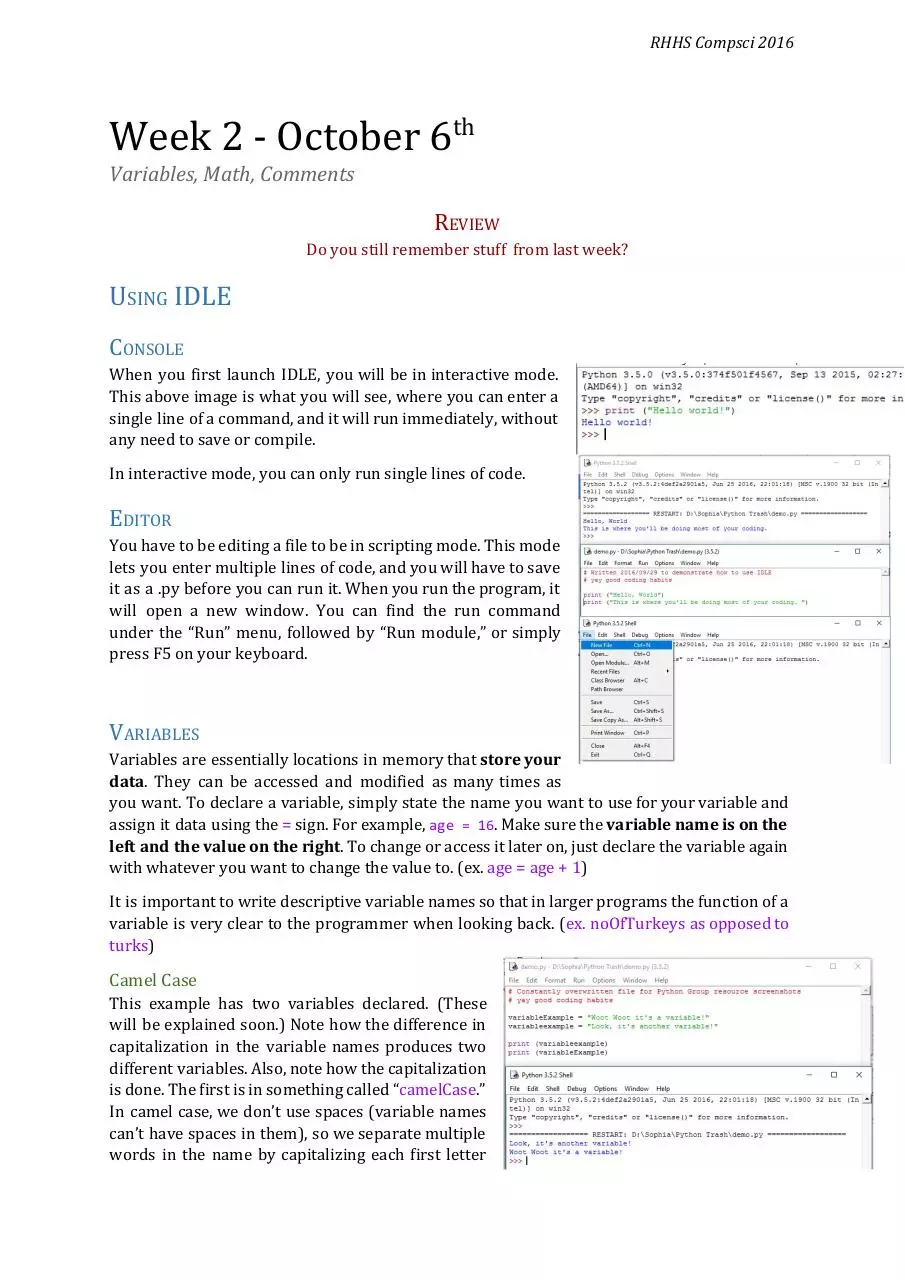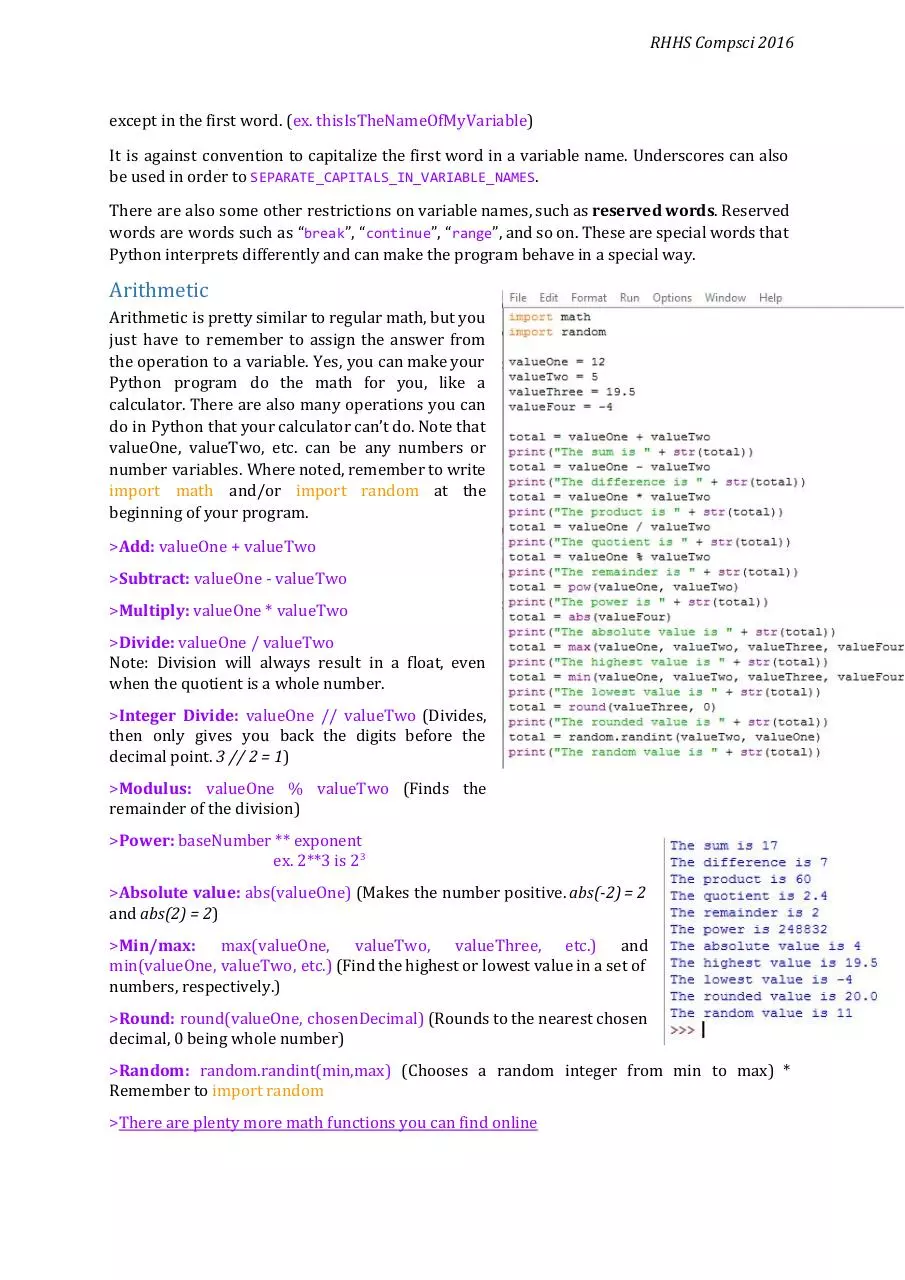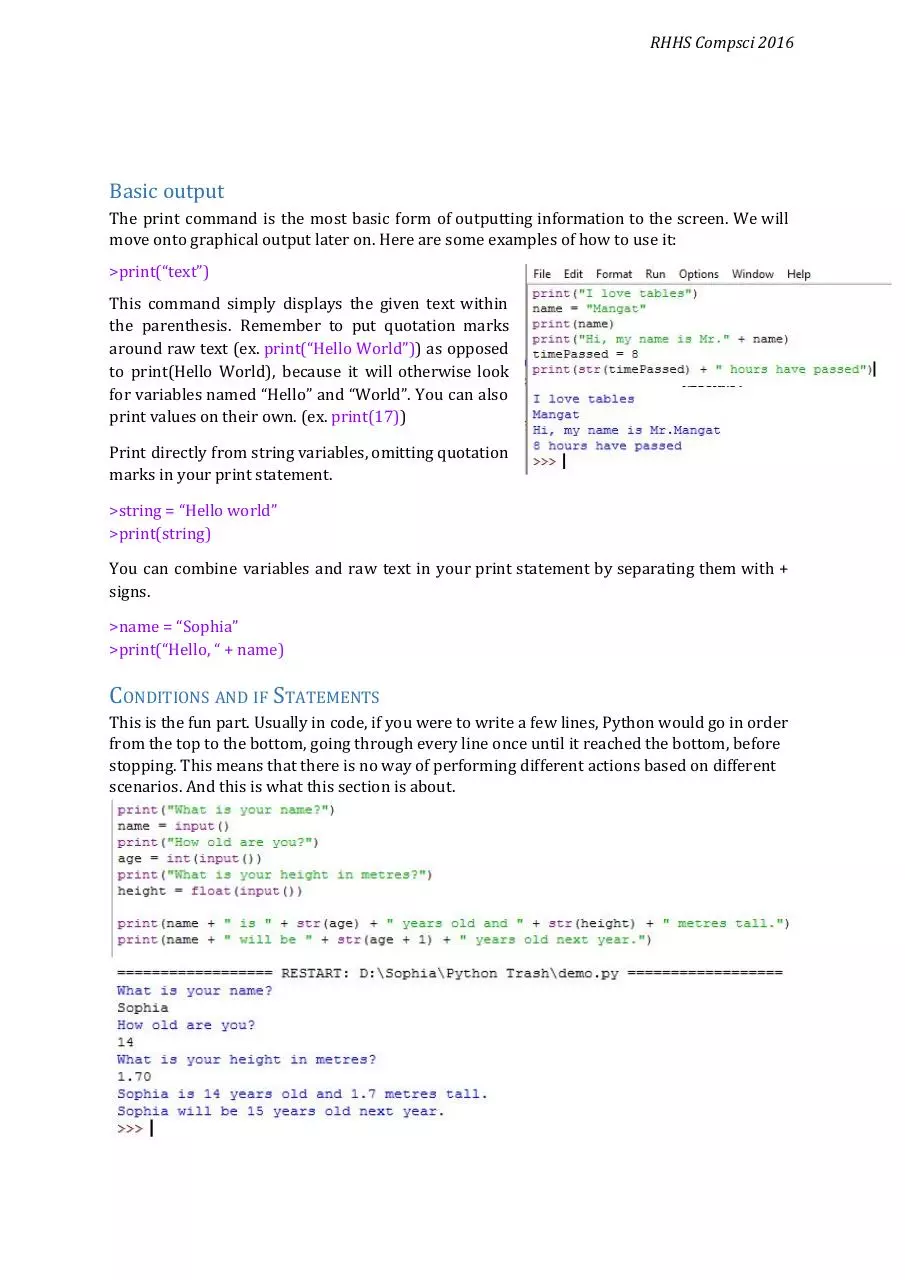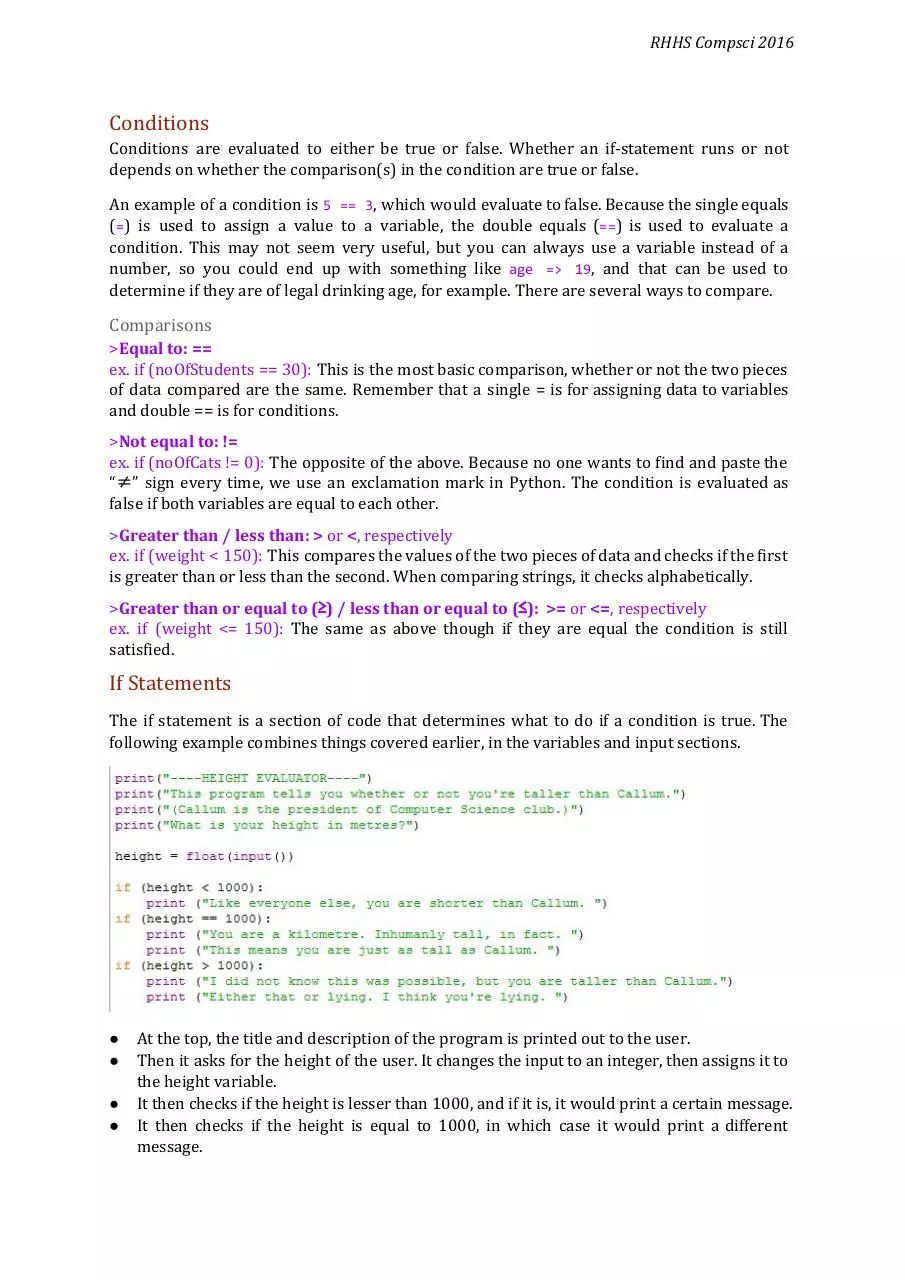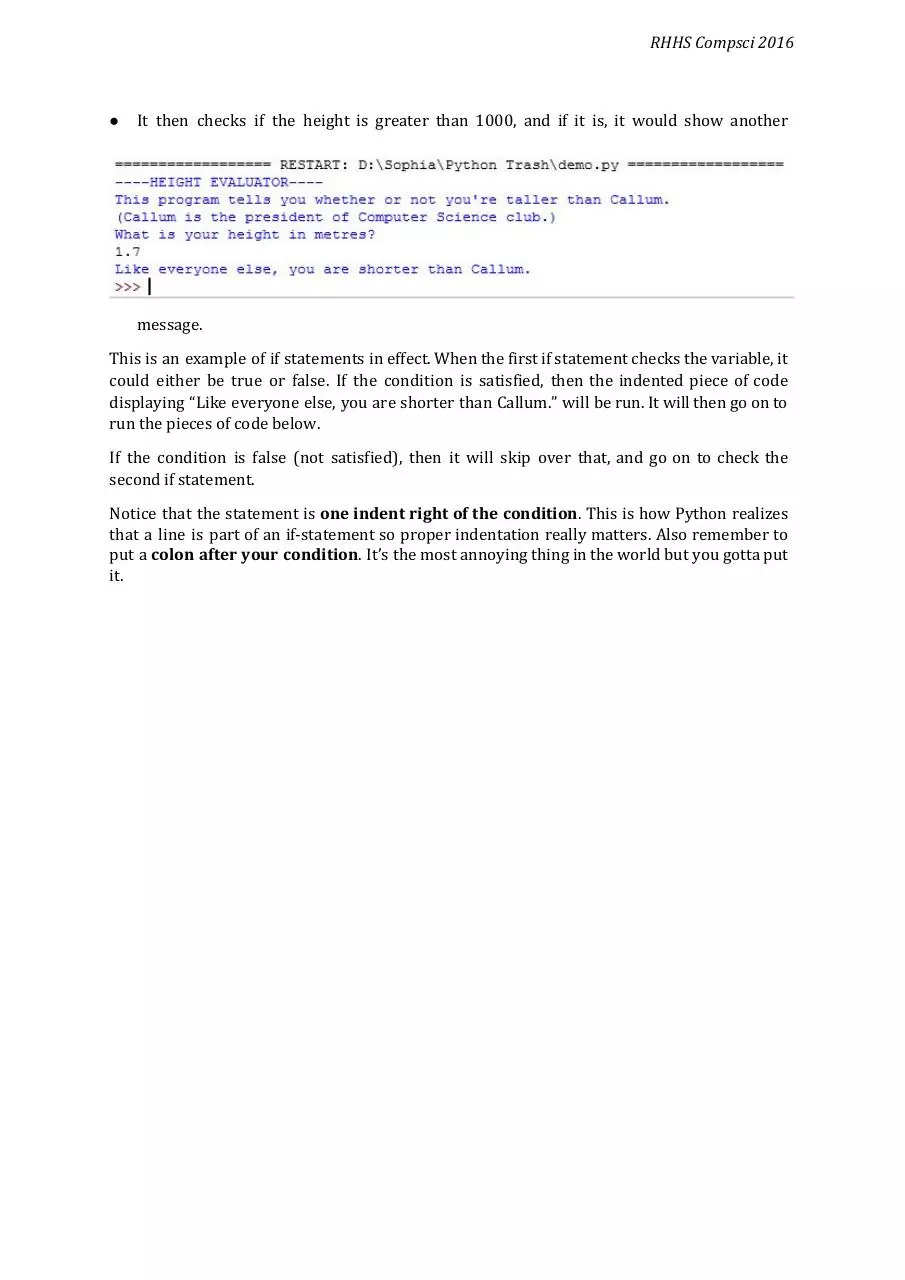### Share on social networks

#### HTML Code

Copy the following HTML code to share your document on a Website or Blog